Next: Compressibility Previous: No Title

Compressible fluids

All real fluids are compressible, and almost all fluids expand when heated. Compression waves can propagate in most fluids: these are the familiar sound waves in the audible frequency range, and ultrasound at higher frequencies. Thermal expansion gives rise to heat convection, especially in the presence of a gravitational field: hot air rises and cold air sinks.

In general, heat transfers and fluid motions are coupled and should be treated together by using the equations of fluid dynamics along with those of thermodynamics and heat diffusion. However, the coupled equations are complicated, and we will start with the simplified assumption that fluid motions occur either isothermally (at constant temperature) or adiabatically (with negligible heat transfer), as a first approximation.

In order to use thermodynamics, it must be possible to define a temperature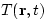that varies with position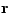and time t, in the same way as one defines other hydrodynamic variables such as the mass density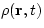, the pressure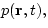and the fluid velocity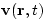. One must be able to consider a volume V that is large enough to be macroscopic (it contains many particles) and small enough to be infinitesimal with respect to variations of T; in addition, the particle velocities within V must be given by the thermal equilibrium distribution, when vieved in a frame moving along with the fluid with the local velocity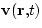.

We will not treat variations in the chemical composition of the fluid, so that in effect we can suppose that the volume V contains N particles of average mass m, and we also assume that the fluid is uncharged and non-magnetic. Then the first law of thermodynamics (just energy balance) can be stated as follows: the heat transfer dQ to the fluid element containing N particles causes a change of the internal energy E and of the volume V according to

 dE = dQ - pdV (1)

An infinitesimal heat transfer dQ corresponds to a change of the entropy S, according to dQ=TdS: thus an adiabatic volume change (dQ=0, no heat transfer) is also isentropic (dS=0). In thermodynamics, the advantage of introducing S is that a system contains a well defined amount of entropy, but not a definite amount of heat (since work can be turned into heat and, to some extent, heat can be used to do work). The entropy S also has the important property that it never decreases for a closed system (second law of thermodynamics). For simplicity, we do not use the second law in this chapter, although we will often refer to the entropy content of the fluid. When we need to carry out a derivation involving S, we assume that the fluid is an ideal gas, work up to the final formula, and then simply quote its general form for any fluid.Next: Compressibility Previous: No Title
Vittorio Celli
11/10/1997